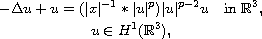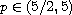Electron. J. Differential Equations, Vol. 2017 (2017), No. 268, pp. 1-18.

### Multiple nodal solutions of nonlinear Choquard equations Zhihua Huang, Jianfu Yang, Weilin Yu

Abstract:
In this article, we consider the existence of multiple nodal solutions of the nonlinear Choquard equationwhere. We show that for any positive integer k, the above problem has at least one radially symmetrical solution changing sign exactly k-times.

Submitted July 15, 2017. Published October 27, 2017.
Math Subject Classifications: 35J61, 35B33, 35B38, 35B65.
Key Words: Nonlinear Choquard equations; nodal solutions; nonlocal term.

Show me the PDF file (292 KB), TEX file for this article.

 Zhihua Huang Department of Mathematics Jiangxi Normal University Nanchang, Jiangxi 330022, China email: zhhuang2016@126.com Jianfu Yang Department of Mathematics Jiangxi Normal University Nanchang, Jiangxi 330022, China email: jfyang_2000@yahoo.com Weilin Yu Department of Mathematics Jiangxi Normal University Nanchang, Jiangxi 330022, China email: williamyu2065@163.com